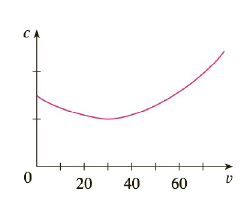Chapter 3.7, Problem 70E

Chapter
Section
Textbook Problem

# The graph shows the fuel consumption c of a car (measured in gallons per hour) as a function of the speed v of the car. At very low speeds the engine runs inefficiently, so initially c decreases as the speed increases. But at high speeds the fuel consumption increases. You can see that c(v) is minimized for this car when v ≈ 30 mi/h. However, for fuel efficiency, what must be minimized is not the consumption in gallons per hour but rather the fuel consumption in gallons per mile. Let’s call this consumption G. Using the graph, estimate the speed at which G has its minimum value.To determine

To estimate:

The speed at which G has its minimum value

Explanation

1) Concept:

The minimum value is obtained when dGdv=0

2) Calculation:

First find the consumption in gallons per mile

c is the fuel consumption of a car given in gallons per hour and v is velocity given in miles per hour

Therefore,

Consumption in gallons per mile that is G given by,

G=cv=gallons per hourmiles per hour=gallonsmiles

To find the minimum calculate dGdv

dGdv=ddv(cv)

By using quotient rule,

dGdv=vddvc-cddv

### Still sussing out bartleby?

Check out a sample textbook solution.

See a sample solution

#### The Solution to Your Study Problems

Bartleby provides explanations to thousands of textbook problems written by our experts, many with advanced degrees!

Get Started

#### f(x) = 3x3 x2 + 10; limxf(x); and limxf(x) x 1 5 10 100 1000 f(x) x 1 5 10 100 1000 f(x)

Applied Calculus for the Managerial, Life, and Social Sciences: A Brief Approach

#### In Problems 7-34, perform the indicated operations and simplify. 26.

Mathematical Applications for the Management, Life, and Social Sciences

#### Multiply: 30,0104080

Elementary Technical Mathematics